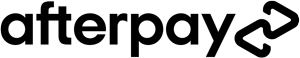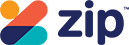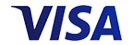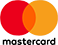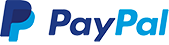Grid List
WAS \$39.95 NOW \$29.95
Increase value Decrease value
WAS \$12.95 NOW \$9.95WAS \$24.95 NOW \$19.95
Increase value Decrease value
WAS \$12.95 NOW \$9.95
Increase value Decrease value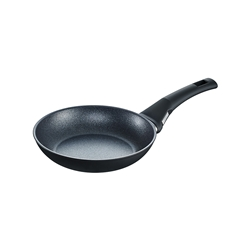WAS \$89.95 NOW \$49.95
Increase value Decrease valueWAS \$49.95 NOW \$39.95
Increase value Decrease value
WAS \$89.95 NOW \$64.95
Increase value Decrease value
WAS \$12.95 NOW \$9.95
Increase value Decrease value
WAS \$12.95 NOW \$9.95
Increase value Decrease value
WAS \$12.95 NOW \$9.95WAS \$24.95 NOW \$19.95
Increase value Decrease value
WAS \$12.95 NOW \$9.95
Increase value Decrease value